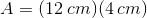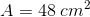## Example Questions

### Example Question #1 : How To Find The Area Of A Parallelogram

In parallelogram, the length of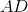isunits, the length of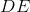isunits, and the length of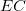isunits.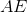is perpendicular fo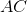. Find the area, in square units, of.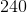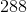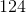Explanation:

The formula to find the area of a parallelogram is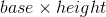The base,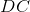, is given by the question.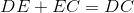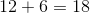You should recognize thatis not only the height of parallelogram, but it is also a leg of the right triangle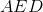.

Use the Pythagorean Theorem to find the length of.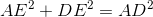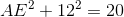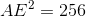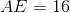Now that we have the height, multiply it by the base to find the area of the parallelogram.

### Example Question #2 : How To Find The Area Of A Parallelogram

A parallelogram has a base of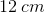and its side is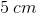long. A line is drawn to connect the edge of the top base with the bottom base. The line is perpendicular to the bottom base, and the base of this triangle is one-fourth the length of the bottom base. Find the area of the parallelogram.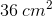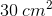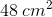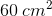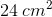Explanation:

The formula for the area of a parallelogram is given by the equation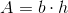, whereis the base andis the height of the parallelogram.

The only given information is that the base is, the side is, and the base of the right triangle in the parallelogram (the triangle formed between the edge of the top base and the bottom base) is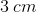because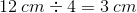.

The last part of information that is required to fulfill the needs of the area formula is the parallelogram's height,. The parallelogram's height is given by the mystery side of the right triangle described in the question. In order to solve for the triangle's third side, we can use the Pythagorean Theorem,.

In this case, the unknown side is one of the legs of the triangle, so we will label it. The given side of the triangle that is part of the base we will call, and the side of the parallelogram is also the hypotenuse of the triangle, so in the Pythagorean Formula its length will be represented by. At this point, we can substitute in these values and solve for: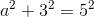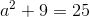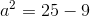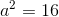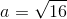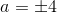, but because we're finding a length, the answer must be 4. The negative option can be negated.

Remembering that we temporarily called"" for the pythagorean theorem, this means that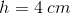.

Now all the necessary parts for the area of a parallelogram equation are available to be used: